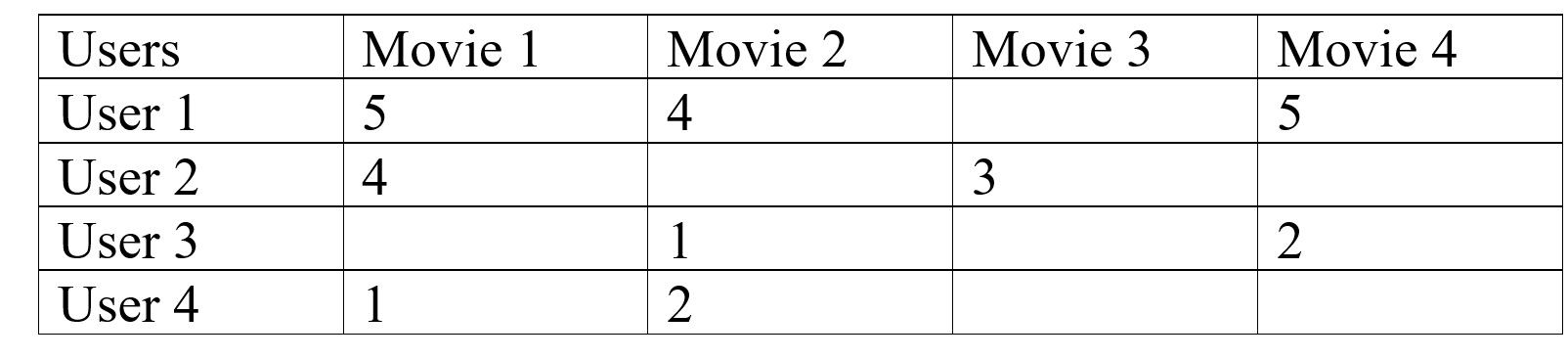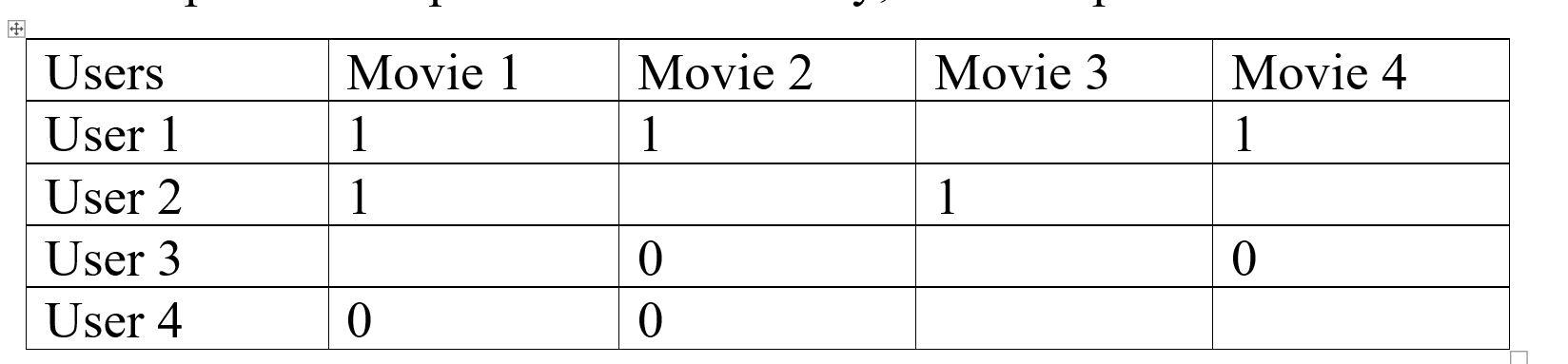Collaborative Filtering – ML

• Last Updated : 03 Jun, 2020

In Collaborative Filtering, we tend to find similar users and recommend what similar users like. In this type of recommendation system, we don’t use the features of the item to recommend it, rather we classify the users into the clusters of similar types, and recommend each user according to the preference of its cluster.

Measuring Similarity:
A simple example of the movie recommendation system will help us in explaining:In this type of scenario, we can see that User 1 and User 2 give nearly similar ratings to the movie, so we can conclude that Movie 3 is also going to be averagely liked by the User 1 but Movie 4 will be a good recommendation to User 2, like this we can also see that there are users who have different choices like User 1 and User 3 are opposite to each other.
One can see that User 3 and User 4 have a common interest in the movie, on that basis we can say that Movie 4 is also going to be disliked by the User 4. This is Collaborative Filtering, we recommend users the items which are liked by the users of similar interest domain.
Cosine Distance:
We can also use the cosine distance between the users to find out the users with similar interests, larger cosine implies that there is a smaller angle between two users, hence they have similar interests.
We can apply the cosine distance between two users in the utility matrix, and we can also give the zero value to all the unfilled columns to make calculation easy, if we get smaller cosine then there will be a larger distance between the users and if the cosine is larger then we have a small angle between the users, and we can recommend them similar things.

Rounding the Data:
In collaborative filtering we round off the data to compare it more easily like we can assign below 3 ratings as 0 and above of it as 1, this will help us to compare data more easily, for example:We again took the previous example and we apply the rounding off process, as you can see how much readable the data has become after performing this process, we can see that User 1 and User 2 are more similar and User 3 and User 4 are more alike.

Normalizing Rating:
In the process of normalizing we take the average rating of a user and subtract all the given ratings from it, so we’ll get either positive or negative values as a rating, which can simply classify further into similar groups. By normalizing the data we can make the clusters of the users which gives a similar rating to similar items and then we can use these clusters to recommend items to the users.

My Personal Notes arrow_drop_up